Related Articles
How to Make ECDF Plot with Seaborn in Python?
• Difficulty Level : Expert
• Last Updated : 24 Jan, 2021

Prerequisites:  Seaborn

In this article, we are going to make the ECDF plot with Seaborn Library.

## ECDF Plot

• ECDF stands for Empirical Commutative Distribution. It is more likely to use instead of the histogram for visualizing the data because the ECDF plot visualizes each and every data point of the dataset directly, which makes it easy for the user to interact with the plot.
• This plot contains more information because it has no bin size setting, which means it doesn’t have any smoothing parameters.
• Since its curves are monotonically increasing, so it is well suited for comparing multiple distributions at the same time.
• In an ECDF plot, the x-axis corresponds to the range of values for the variable whereas the y-axis corresponds to the proportion of data points that are less than or equal to the corresponding value of the x-axis.
• We can make the ECDF plot directly by using ecdfplot() function, or we can also make the plot by using displot() function with the new Seaborn version.

### Installation:

To install the Seaborn library, write the following command in your command prompt.

`pip install seaborn`

This ECDF plot and displot() function is available only in the new version of Seaborn that is version 0.11.0 or above. If already install Seaborn upgrade it by writing the following command.

`pip install seaborn==0.11.0`

For a better understanding of the ECDF plot. Let’s plot and do some examples using the datasets.

### Step-by-Step Approach:

• Import the seaborn library.
• Create or load the dataset from the seaborn library.
• Select the column for which you are plotting the ECDF plot.
• For plotting the ECDF plot there are two ways are as follows:
• The first way is to use ecdfplot() function to directly plot the ECDF plot and in the function pass you data and column name on which you are plotting.

Syntax:

seaborn.ecdfplot(data=’dataframe’,x=’column_name’,y=’column_name’, hue=’color_column’)

• The second way is to use displot() function and pass your data and column on which you are making the plot and pass the parameter of displot kind=’ecdf’.

Syntax:

seaborn.displot(data=’dataframe’, x=’column_name’,y=’column_name’ kind=’type_of_plot’,hue=’color_column’, palette=’color’

The below table shows the list of parameters used in this article.

Method 1: Using ecdfplot() method

In this method, we are using ‘excerise’ data provided by seaborn.

## Python

 `# importing library``import` `seaborn as sns`` ` `# loading exercise dataset provided by seaborn``excr ``=` `sns.load_dataset(``'exercise'``)`` ` `# printing the dataset``print``(excr)`

Output: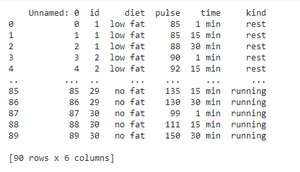Example 1: Making ECDF plot by using exercise dataset provided by seaborn.

## Python

 `# importing libraries``import` `seaborn as sns``import` `matplotlib.pyplot as plt`` ` `# loading exercise dataset provided by seaborn``excr ``=` `sns.load_dataset(``'exercise'``)`` ` `# making ECDF plot ``sns.ecdfplot(data``=``excr,x``=``'pulse'``)`` ` `# visualizing the plot using matplotlib.pyplot ``# show() function``plt.show()`

Output: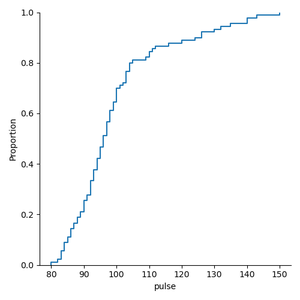Example 2: Making ECDF plot by interchanging the plot axis.

## Python

 `# importing libraries``import` `seaborn as sns``import` `matplotlib.pyplot as plt`` ` `# loading exercise dataset provided by seaborn``excr ``=` `sns.load_dataset(``'exercise'``)`` ` `# making ECDF plot ``sns.ecdfplot(data``=``excr,y``=``'pulse'``)`` ` `# visualizing the plot using matplotlib.pyplot``# show() function``plt.show()`

Output: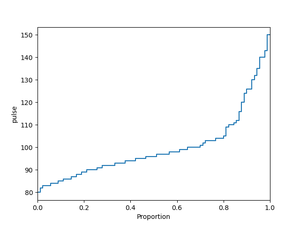Example 3: Making ECDF plot when we have multiple distributions.

## Python

 `# importing libraries``import` `seaborn as sns``import` `matplotlib.pyplot as plt`` ` `# loading exercise dataset provided by seaborn``excr ``=` `sns.load_dataset(``'exercise'``)`` ` `# making ECDF plot when we have multiple ``# distributions``sns.ecdfplot(data``=``excr, x``=``'pulse'``, hue``=``'kind'``)`` ` `# visualizing the plot using matplotlib.pyplot ``# show() function``plt.show()`

Output: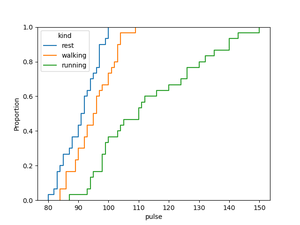The above plot shows the distribution of pulse rate of the peoples with respect to the kind i.e, rest, walking, running.

Method 2: Using displot() method

In this method, we are using ‘diamonds’ data provided by seaborn.

## Python

 `# importing library``import` `seaborn as sns`` ` `# loading diamonds dataset provided by seaborn``diam ``=` `sns.load_dataset(``'diamonds'``)`` ` `# printing the dataset``print``(diam)`

Output: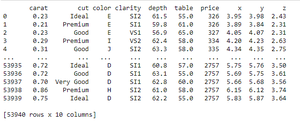Example 1: Plotting ECDF plot using displot() on penguins dataset provided by seaborn.

## Python

 `# importing libraries``import` `seaborn as sns``import` `matplotlib.pyplot as plt`` ` `# loading diamonds dataset provided by seaborn``diam ``=` `sns.load_dataset(``'diamonds'``)`` ` `# making ECDF plot using displot() on depth ``# of the diamonds``sns.displot(data``=``diam,x``=``'depth'``,kind``=``'ecdf'``)`` ` `# visualizing the plot using matplotlib.pyplot ``# show() function``plt.show()`

Output: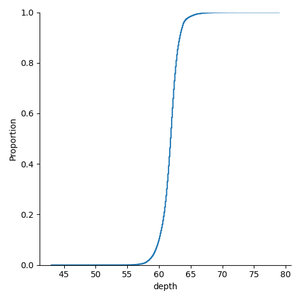Example 2: Plotting ECDF plot using displot() when we have multiple distributions with default setting.

## Python

 `# importing libraries``import` `seaborn as sns``import` `matplotlib.pyplot as plt`` ` `# loading diamonds dataset provided by seaborn``diam ``=` `sns.load_dataset(``'diamonds'``)`` ` `# making ECDF plot using displot() on depth``# of the diamond on the basis of cut``sns.displot(data``=``diam,x``=``'depth'``,kind``=``'ecdf'``,hue``=``'cut'``)`` ` `# visualizing the plot using matplotlib.pyplot``# show() function``plt.show()`

Output: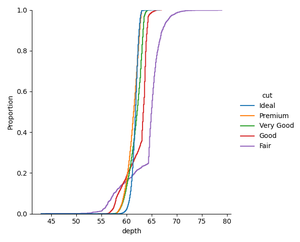The above plot shows the depth of the diamonds on the basis of their cut.

Example 3: Making ECDF plot using displot() by setting up the color.

## Python

 `# importing libraries``import` `seaborn as sns``import` `matplotlib.pyplot as plt`` ` `# loading diamonds dataset provided by seaborn``diam ``=` `sns.load_dataset(``'diamonds'``)`` ` `# making ECDF plot using displot() on table ``# column on the basis of cut of diamond``# setting up the color of plot by setting``# up the palette to icefire_r``sns.displot(data``=``diam,x``=``'table'``,kind``=``'ecdf'``,hue``=``'cut'``,palette``=``'icefire_r'``)`` ` `# visualizing the plot using matplotlib.pyplot``# show() function``plt.show()`

Output: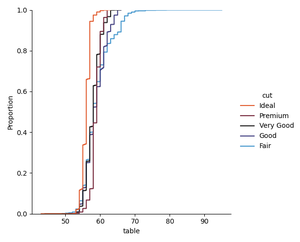We can set the palette to Accent_r, magma_r, plasma, plasma_r, etc, according to our choice, it has many other options available.

Attention geek! Strengthen your foundations with the Python Programming Foundation Course and learn the basics.

To begin with, your interview preparations Enhance your Data Structures concepts with the Python DS Course.

My Personal Notes arrow_drop_up